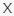# Fun Color Vision Challenge VideoQuizStar

Q 1. Which picture matches the one on the left?Choices:

A
B
C
D

B

Q 2. Which picture matches the one on the left?Choices:

A
B
C
D

A

Q 3. Which one matches the one on the left?Choices:

A
B
C
D

D

Q 4. Do you know which one matches the picture on the left?Choices:

A
B
C
D

C

Q 5. Can you see which color is different?Choices:

A
B
C

B

Q 6. Which color is different here?Choices:

A
B
C

B

Q 7. Which number do you see here?Choices:

31
11
34
74

74

Q 8. What do you see in this picture?Choices:

Frog
Fish
Boat
Clam

Fish

Q 9. Which number do you see?Choices:

2
4
3
7

2

Q 10. Which numeral is fainter?Choices:

4
2
Neither

2

Q 11. Apart from color, are these two images identical?Choices:

No
Yes

Yes

Q 12. How many numerals can you see in this picture?Choices:

I don’t see any
3
4
6

4

Q 13. Which images feature the same numbers?Choices:

A/C and A/D
A/D and B/C
A/B and C/D
They are all different

A/D and B/C

Q 14. What colour is the object in the circle?Choices:

Grey
Red
Blue
Green

Blue

Q 15. What do you recognise here?Choices:

Giraffe
Horse
Llama
Dinosaur

Giraffe

Q 16. How many shades can you see in the winding line?Choices:

1
2
3
4

4

Q 17. Where is the opening in the circle pointing?Choices:

A
B
C
D

A

Q 18. Which one is different?Choices:

A
B
C

C

Q 19. Which two colors make up the color on the left?Choices:

A
B
C
D

C

Q 20. Which two colors make up the color on the left?Choices:

A
B
C
D

B

Q 21. Getting tricky now! Which two colors make up the color on the left?Choices:

A
B
C
D

C

Q 22. How many squares have a different color?Choices:

1
2
3
4

2

Q 23. Let’s see if you can match which one fits the picture on the left!Choices:

A
B
C
D

A

Q 24. What do you see here?Choices:

92
63
82
52

52

Q 25. In which row can you find a different colored square?Choices:

A
B
C

C

Q 26. Which of these blues is closer to purple?Choices:

A
B

B

Q 27. Are 5 and 6 the same?Choices:

Yes
No

No

Q 28. What color would you get if you mixed 3 and 7?Choices:

Grey
Navy
Cyan
Maroon

Maroon

Q 29. Which orange is lighter?Choices:

A
B

B

Q 30. Which green is more saturated?Choices:

A
B